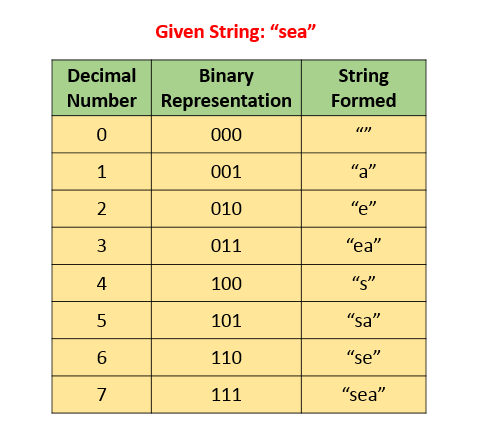## Solution

#### Approach 1: Brute Force

Algorithm

The idea behind this approach is as follows. We create a list of all the possible strings that can be formed by deleting one or more characters from the given string . In order to do so, we make use of a recursive function generate(s, str, i, l) which creates a string by adding and by removing the current character() from the string to the string formed till the index . Thus, it adds the character to and calls itself as generate(s, str + s.charAt(i), i + 1, l). It also omits the character to and calls itself as generate(s, str, i + 1, l).

Thus, at the end the list contains all the required strings that can be formed using . Then, we look for the strings formed in into the dictionary available to see if a match is available. Further, in case of a match, we check for the length of the matched string to maximize the length and we also take care to consider the lexicographically smallest string in case of length match as well.

Complexity Analysis

• Time complexity : . generate calls itself times. Here, refers to the length of string .

• Space complexity : . List contains strings.

#### Approach 2: Iterative Brute Force

Algorithm

Instead of using recursive generate to create the list of possible strings that can be formed using by performing delete operations, we can also do the same process iteratively. To do so, we use the concept of binary number generation.

We can treat the given string along with a binary represenation corresponding to the indices of . The rule is that the character at the position has to be added to the newly formed string only if there is a boolean 1 at the corresponding index in the binary representation of a number currently considered.

We know a total of such binary numbers are possible if there are positions to be filled( also corresponds to the number of characters in ). Thus, we consider all the numbers from to in their binary representation in a serial order and generate all the strings possible using the above rule.

The figure below shows an example of the strings generated for the given string :"sea".A problem with this method is that the maximum length of the string can be 32 only, since we make use of an integer and perform the shift operations on it to generate the binary numbers.

Complexity Analysis

• Time complexity : . strings are generated.

• Space complexity : . List contains strings.

#### Approach 3: Sorting and Checking Subsequence

Algorithm

The matching condition in the given problem requires that we need to consider the matching string in the dictionary with the longest length and in case of same length, the string which is smallest lexicographically. To ease the searching process, we can sort the given dictionary's strings based on the same criteria, such that the more favorable string appears earlier in the sorted dictionary.

Now, instead of performing the deletions in , we can directly check if any of the words given in the dictionary(say ) is a subsequence of the given string , starting from the beginning of the dictionary. This is because, if is a subsequence of , we can obtain by performing delete operations on .

If is a subsequence of every character of will be present in . The following figure shows the way the subsequence check is done for one example:

!?!../Documents/524_Longest_Word.json:1000,563!?!

As soon as we find any such , we can stop the search immediately since we've already processed to our advantage.

Complexity Analysis

• Time complexity : . Here refers to the number of strings in list and refers to average string length. Sorting takes and isSubsequence takes to check whether a string is a subsequence of another string or not.

• Space complexity : . Sorting takes space in average case.

#### Approach 4: Without Sorting

Algorithm

Since sorting the dictionary could lead to a huge amount of extra effort, we can skip the sorting and directly look for the strings in the unsorted dictionary such that is a subsequence in . If such a string is found, we compare it with the other matching strings found till now based on the required length and lexicographic criteria. Thus, after considering every string in , we can obtain the required result.

Complexity Analysis

• Time complexity : . One iteration over all strings is required. Here refers to the number of strings in list and refers to average string length.

• Space complexity : . variable is used.

Analysis written by: @vinod23